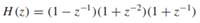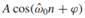# Suppose that a system is defined by the system function (a) Write the time-domain description of…

Suppose that a system is defined by the system function(a) Write the time-domain description of this system in the form of a difference equation.

(b) Write a formula for the frequency response of the system.

(c) Derive simple formulas for the magnitude response versusωˆ and the phase response versus ωˆ. These formulas must contain no complex terms and no square roots.

(d) This system can “null” certain input signals. For which input frequencies ωˆ 0 is the response to x[n] = cos(ωˆ 0n) equal to zero?

(e) When the input to the system is x[n] = cos(πn/3), determine the output signal y[n] in the form:Give numerical values for the constants A, ωˆ 0, and ϕ.